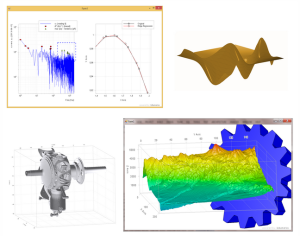# Drawing 3D graphs in C# with ILNumericsThis article deals with how elaborate graphs can be drawn in C# with the help of the ILNumerics class libraries – if requested the graphs can even be animated as well.
Similar to all of my articles on this blog, I will briefly introduce the library first, then I will obtain the necessary bases which are needed for the coding later on and finally I will show you step by step how to get there. Let’s start!
What is ILNumerics?
ILNumerics is a .NET class library that includes a number of components. The two core components are the Compute Engine and the Visualization Engine. ILNumerics expands the .NET-Framework by complex numbers, scalars and vector calculations, matrices and matrix operations, multi-dimensional data structures and much more.
Another advantage of ILNumerics is the Visualization Engine that makes plotting of two or three-dimensional […]